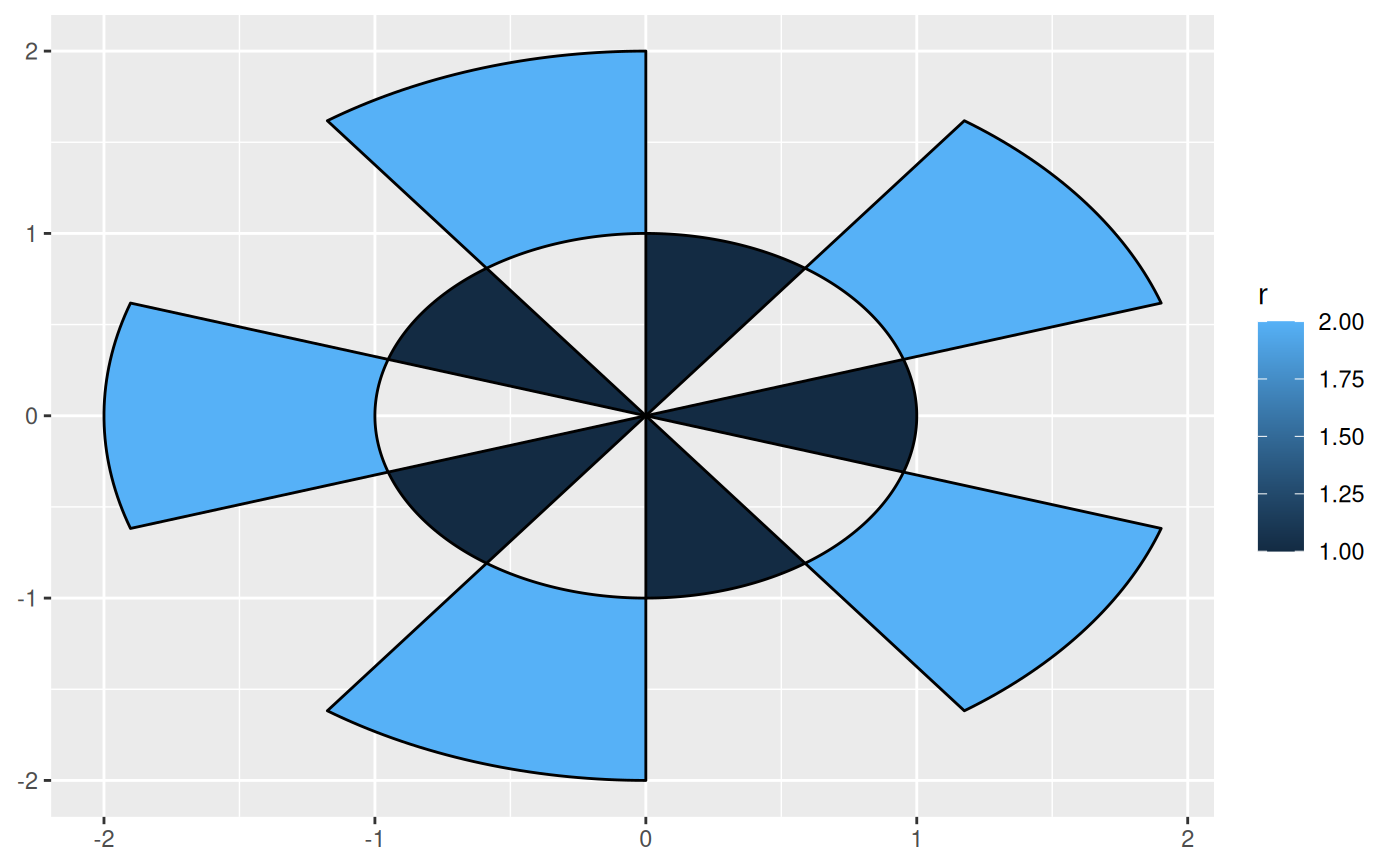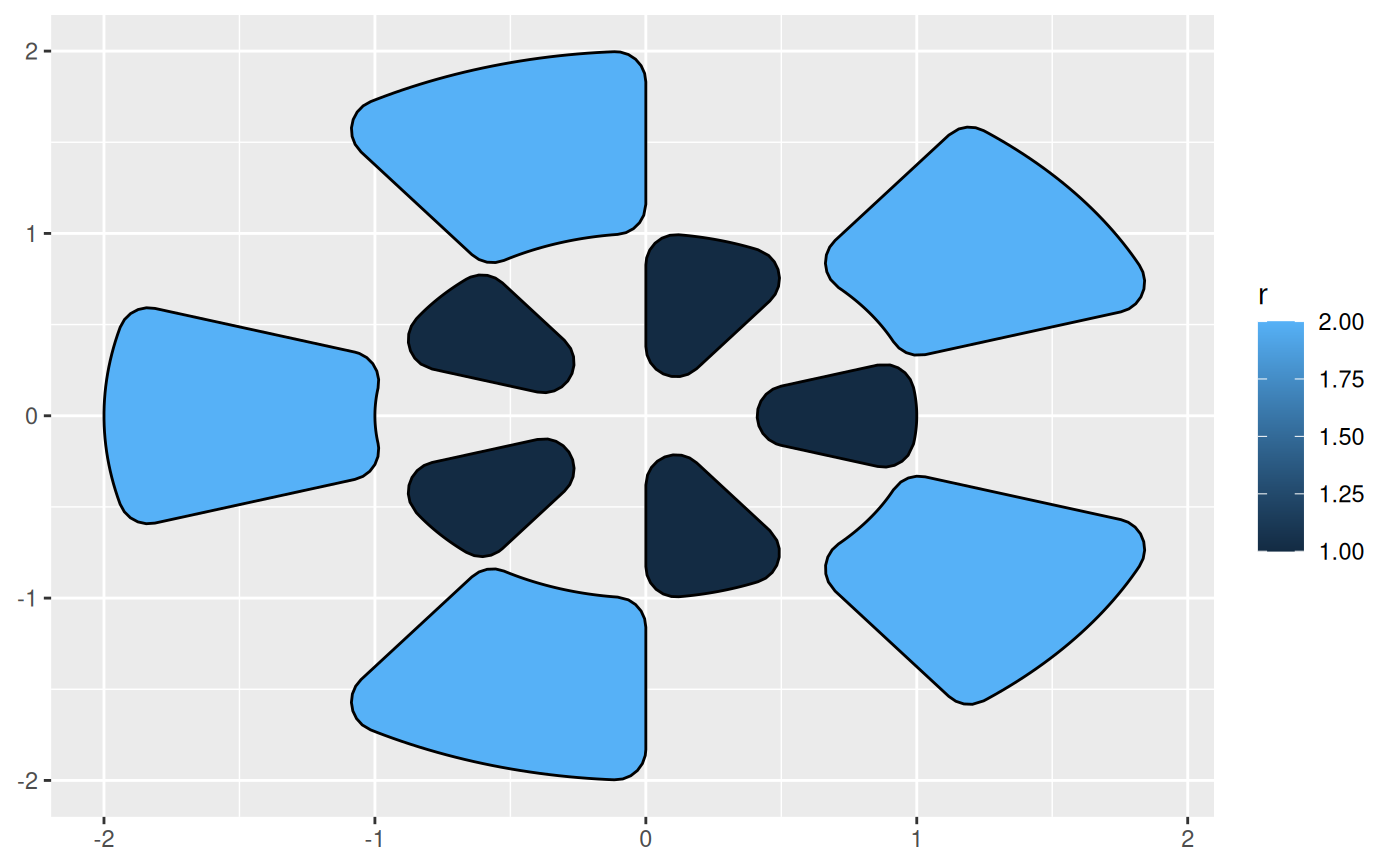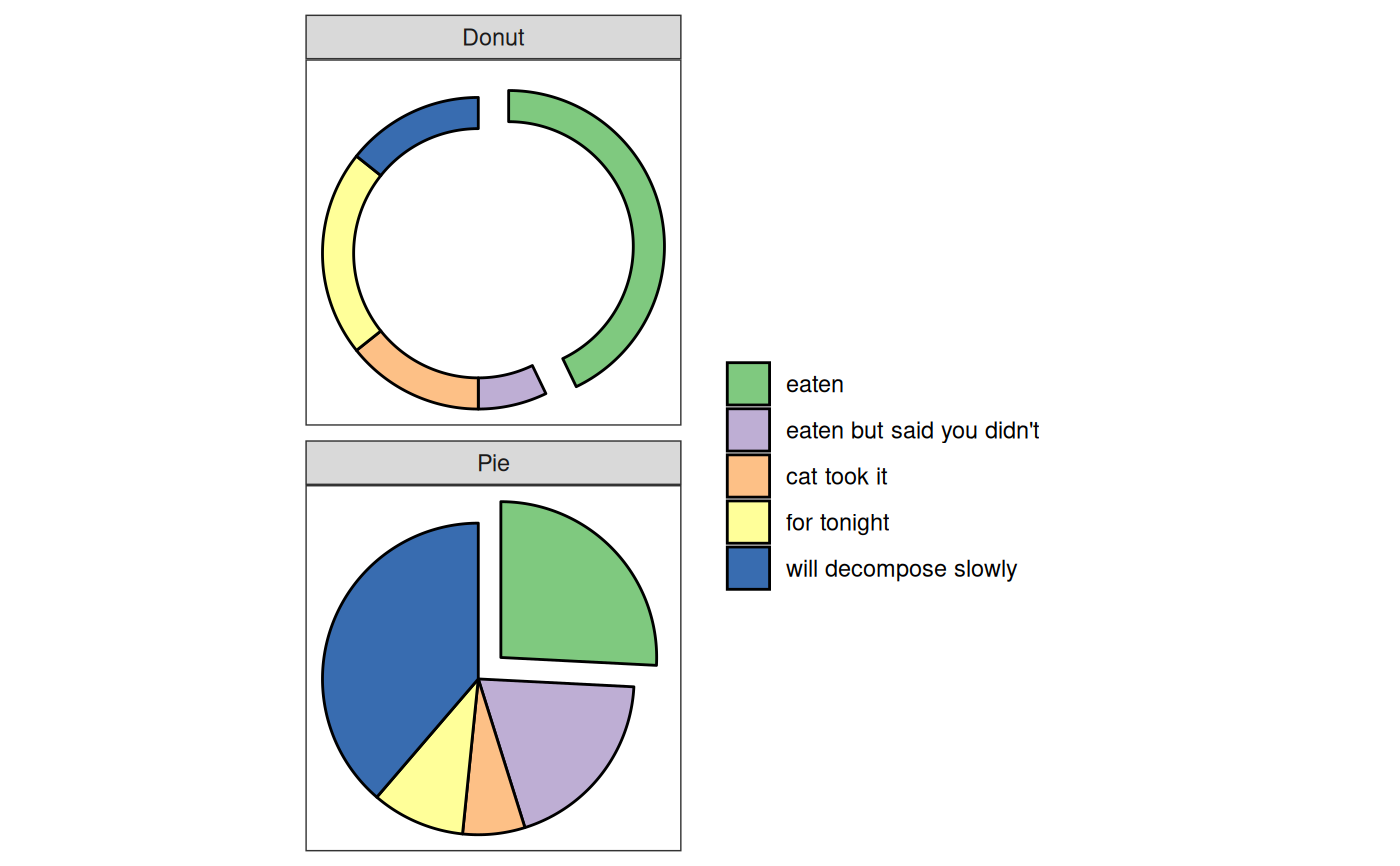This set of stats and geoms makes it possible to draw arcs and wedges as known from pie and donut charts as well as more specialized plottypes such as sunburst plots.

stat_arc_bar(
mapping = NULL,
data = NULL,
geom = "arc_bar",
position = "identity",
n = 360,
na.rm = FALSE,
show.legend = NA,
inherit.aes = TRUE,
...
)

stat_pie(
mapping = NULL,
data = NULL,
geom = "arc_bar",
position = "identity",
n = 360,
sep = 0,
na.rm = FALSE,
show.legend = NA,
inherit.aes = TRUE,
...
)

geom_arc_bar(
mapping = NULL,
data = NULL,
stat = "arc_bar",
position = "identity",
n = 360,
expand = 0,
na.rm = FALSE,
show.legend = NA,
inherit.aes = TRUE,
...
)

## Arguments

mapping

Set of aesthetic mappings created by aes(). If specified and inherit.aes = TRUE (the default), it is combined with the default mapping at the top level of the plot. You must supply mapping if there is no plot mapping.

data

The data to be displayed in this layer. There are three options:

If NULL, the default, the data is inherited from the plot data as specified in the call to ggplot().

A data.frame, or other object, will override the plot data. All objects will be fortified to produce a data frame. See fortify() for which variables will be created.

A function will be called with a single argument, the plot data. The return value must be a data.frame, and will be used as the layer data. A function can be created from a formula (e.g. ~ head(.x, 10)).

geom

The geometric object to use to display the data, either as a ggproto Geom subclass or as a string naming the geom stripped of the geom_ prefix (e.g. "point" rather than "geom_point")

position

Position adjustment, either as a string naming the adjustment (e.g. "jitter" to use position_jitter), or the result of a call to a position adjustment function. Use the latter if you need to change the settings of the adjustment.

n

The number of points used to draw a full circle. The number of points on each arc will then be calculated as n / span-of-arc

na.rm

If FALSE, the default, missing values are removed with a warning. If TRUE, missing values are silently removed.

show.legend

logical. Should this layer be included in the legends? NA, the default, includes if any aesthetics are mapped. FALSE never includes, and TRUE always includes. It can also be a named logical vector to finely select the aesthetics to display.

inherit.aes

If FALSE, overrides the default aesthetics, rather than combining with them. This is most useful for helper functions that define both data and aesthetics and shouldn't inherit behaviour from the default plot specification, e.g. borders().

...

Other arguments passed on to layer(). These are often aesthetics, used to set an aesthetic to a fixed value, like colour = "red" or size = 3. They may also be parameters to the paired geom/stat.

sep

The separation between arcs in pie/donut charts

stat

The statistical transformation to use on the data for this layer, either as a ggproto Geom subclass or as a string naming the stat stripped of the stat_ prefix (e.g. "count" rather than "stat_count")

expand

A numeric or unit vector of length one, specifying the expansion amount. Negative values will result in contraction instead. If the value is given as a numeric it will be understood as a proportion of the plot area width.

As expand but specifying the corner radius.

## Details

An arc bar is the thick version of an arc; that is, a circle segment drawn as a polygon in the same way as a rectangle is a thick version of a line. A wedge is a special case of an arc where the inner radius is 0. As opposed to applying coord_polar to a stacked bar chart, these layers are drawn in cartesian space, which allows for transformations not possible with the native ggplot2 approach. Most notable of these are the option to explode arcs and wedgets away from their center point, thus detaching it from the main pie/donut.

## Aesthetics

geom_arc_bar understand the following aesthetics (required aesthetics are in bold):

• x0

• y0

• r0

• r

• start - when using stat_arc_bar

• end - when using stat_arc_bar

• amount - when using stat_pie

• explode

• color

• fill

• linewidth

• linetype

• alpha

## Computed variables

x, y

x and y coordinates for the polygon

x, y

The start coordinates for the segment

geom_arc() for drawing arcs as lines

## Examples

# If you know the angle spans to plot it is easy
arcs <- data.frame(
start = seq(0, 2 * pi, length.out = 11)[-11],
end = seq(0, 2 * pi, length.out = 11)[-1],
r = rep(1:2, 5)
)

# Behold the arcs
ggplot(arcs) +
geom_arc_bar(aes(x0 = 0, y0 = 0, r0 = r - 1, r = r, start = start,
end = end, fill = r))# geom_arc_bar uses geom_shape to draw the arcs, so you have all the
# possibilities of that as well, e.g. rounding of corners
ggplot(arcs) +
geom_arc_bar(aes(x0 = 0, y0 = 0, r0 = r - 1, r = r, start = start,
end = end, fill = r), radius = unit(4, 'mm'))# If you got values for a pie chart, use stat_pie
states <- c(
'eaten', "eaten but said you didn\'t", 'cat took it', 'for tonight',
'will decompose slowly'
)
pie <- data.frame(
state = factor(rep(states, 2), levels = states),
type = rep(c('Pie', 'Donut'), each = 5),
r0 = rep(c(0, 0.8), each = 5),
focus = rep(c(0.2, 0, 0, 0, 0), 2),
amount = c(4, 3, 1, 1.5, 6, 6, 1, 2, 3, 2)
)

# Look at the cakes
ggplot() + geom_arc_bar(aes(
x0 = 0, y0 = 0, r0 = r0, r = 1, amount = amount,
fill = state, explode = focus
),
data = pie, stat = 'pie'
) +
facet_wrap(~type, ncol = 1) +
coord_fixed() +
theme_no_axes() +
scale_fill_brewer('', type = 'qual')